library(DATA606)
## Loading required package: shiny
## Loading required package: openintro
## Please visit openintro.org for free statistics materials
##
## Attaching package: 'openintro'
## The following objects are masked from 'package:datasets':
##
##     cars, trees
## Loading required package: OIdata
## Loading required package: RCurl
## Loading required package: bitops
## Loading required package: maps
## Loading required package: ggplot2
##
## Attaching package: 'ggplot2'
## The following object is masked from 'package:openintro':
##
##     diamonds
## Loading required package: markdown
##
## Welcome to CUNY DATA606 Statistics and Probability for Data Analytics
## This package is designed to support this course. The text book used
## is OpenIntro Statistics, 3rd Edition. You can read this by typing
## vignette('os3') or visit www.OpenIntro.org.
##
## The getLabs() function will return a list of the labs available.
##
## The demo(package='DATA606') will list the demos that are available.
##
## Attaching package: 'DATA606'
## The following object is masked from 'package:utils':
##
##     demo

Area under the curve, Part I. (4.1, p. 142) What percent of a standard normal distribution $$N(\mu=0, \sigma=1)$$ is found in each region? Be sure to draw a graph.

## (a) $$Z < -1.35$$

mu <- 0
sd <- 1
Z <- -1.35
x <- Z * sd + mu
pnorm(x, mean = 0, sd = 1)
##  0.08850799
# Probability curve plot
normalPlot(mean = 0, sd = 1, bounds = c(-Inf, x), tails = FALSE)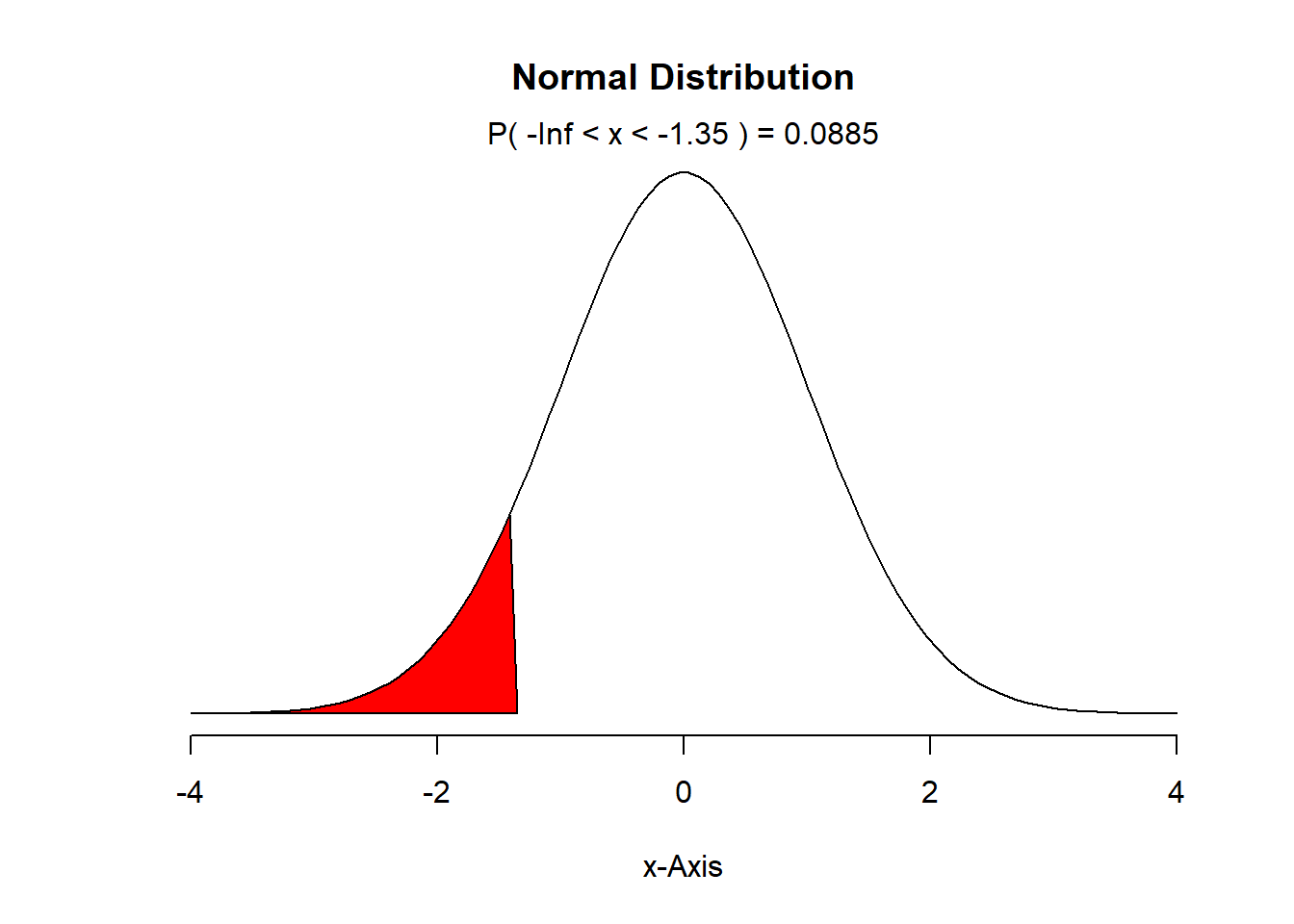The percent of a standard normal distribution found in this region is: 8.85%

## (b) $$Z > 1.48$$

mu <- 0
sd <- 1
Z <- 1.48
x <- Z * sd + mu

#P(Z > 1.48) = 1 - P(Z<1.48)
1 - pnorm(Z)
##  0.06943662
# Probability curve plot
normalPlot(mean = 0, sd = 1, bounds = c(x, Inf), tails = FALSE)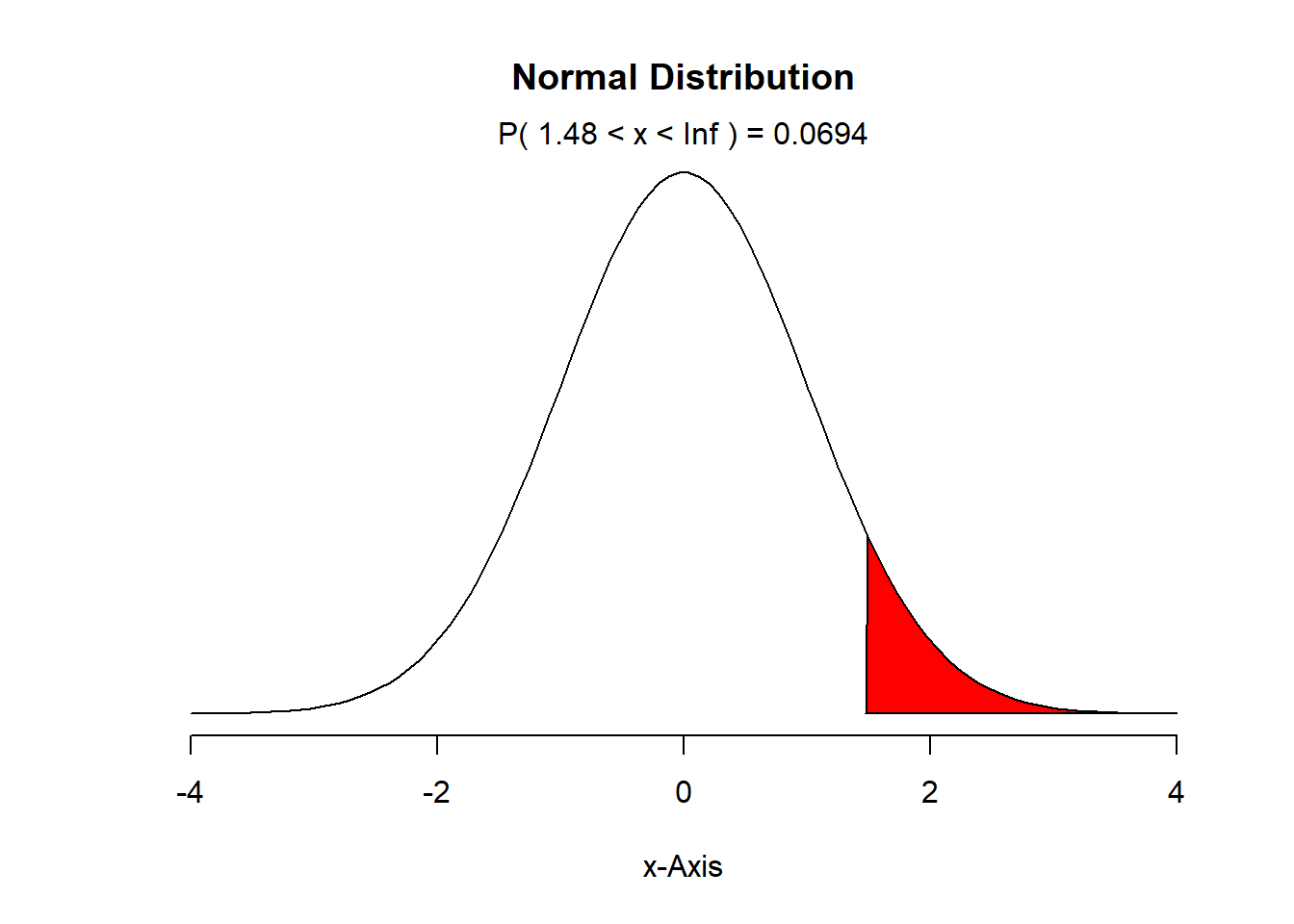The percent of a standard normal distribution found in this region is: 6.94%

## (c) $$-0.4 < Z < 1.5$$

# P(−0.4<Z<1.5) = P(Z<1.5) - P(Z<-0.4)
mu <- 0
sd <- 1
Z1 <- -0.4
Z2 <- 1.5

x1 <- Z1 * sd + mu
x2 <- Z2 * sd + mu

p1 <- pnorm(x1, mean = 0, sd = 1)
p2 <- pnorm(x2, mean = 0, sd = 1)
p2 - p1
##  0.5886145
# Probability curve plot
normalPlot(mean = 0, sd = 1, bounds = c(x1, x2), tails = FALSE)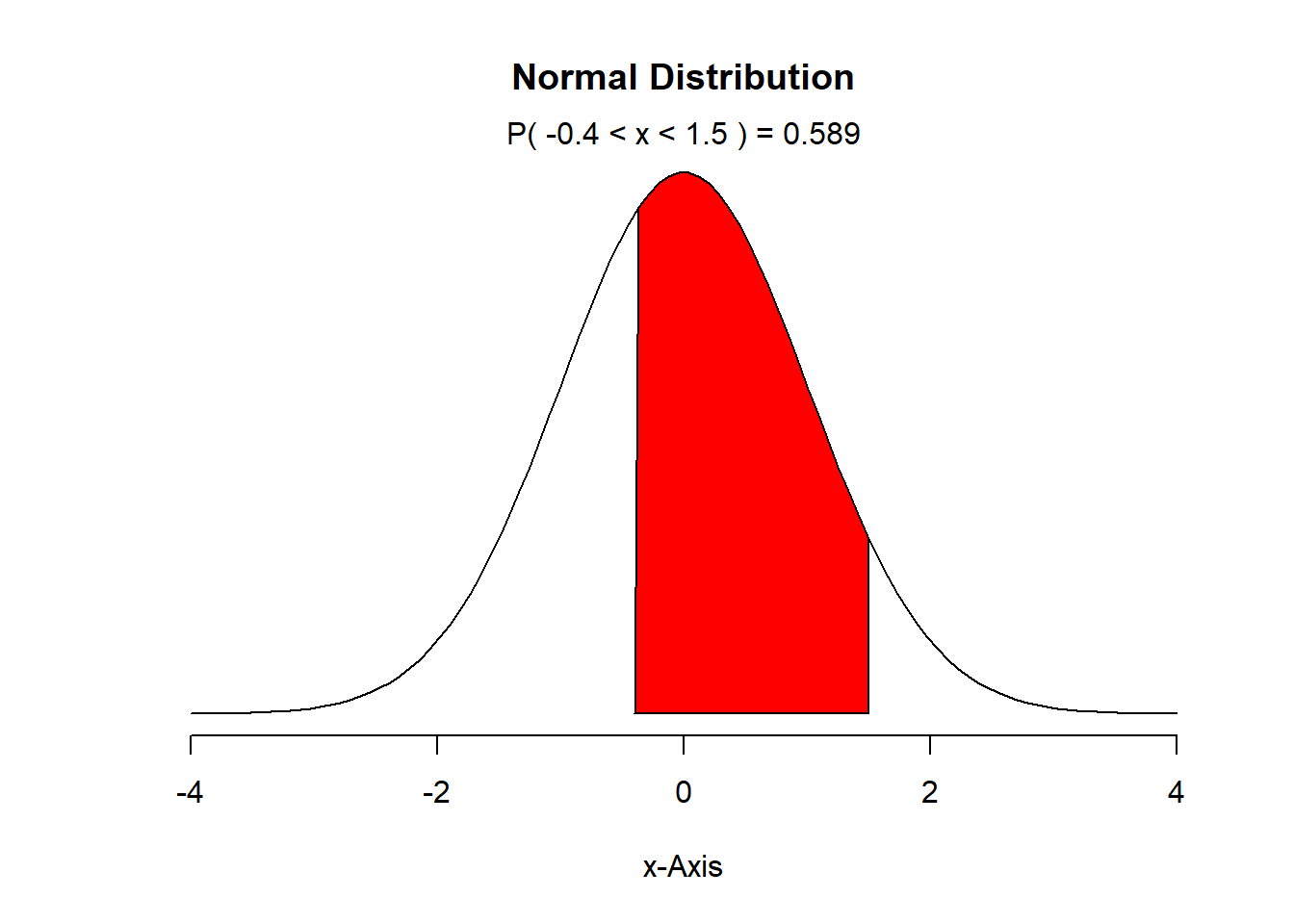The percent of a standard normal distribution found in this region is: 58.9%

## (d) $$|Z| > 2$$

# |Z| > 2 ==> Z < -2 or Z > 2
# so P(|Z|>2) = P(Z < -2 or Z > 2) = P(Z< -2) + P(Z > 2) = P(Z< -2) + ( 1 - P(Z < 2))

#P(Z< -2) + ( 1 - P(Z < 2))
pnorm(-2, mean = 0, sd = 1) + 1 - pnorm(2, mean = 0, sd = 1)
##  0.04550026
# Probability curve plot
normalPlot(mean = 0, sd = 1, bounds = c(-2, 2), tails = TRUE)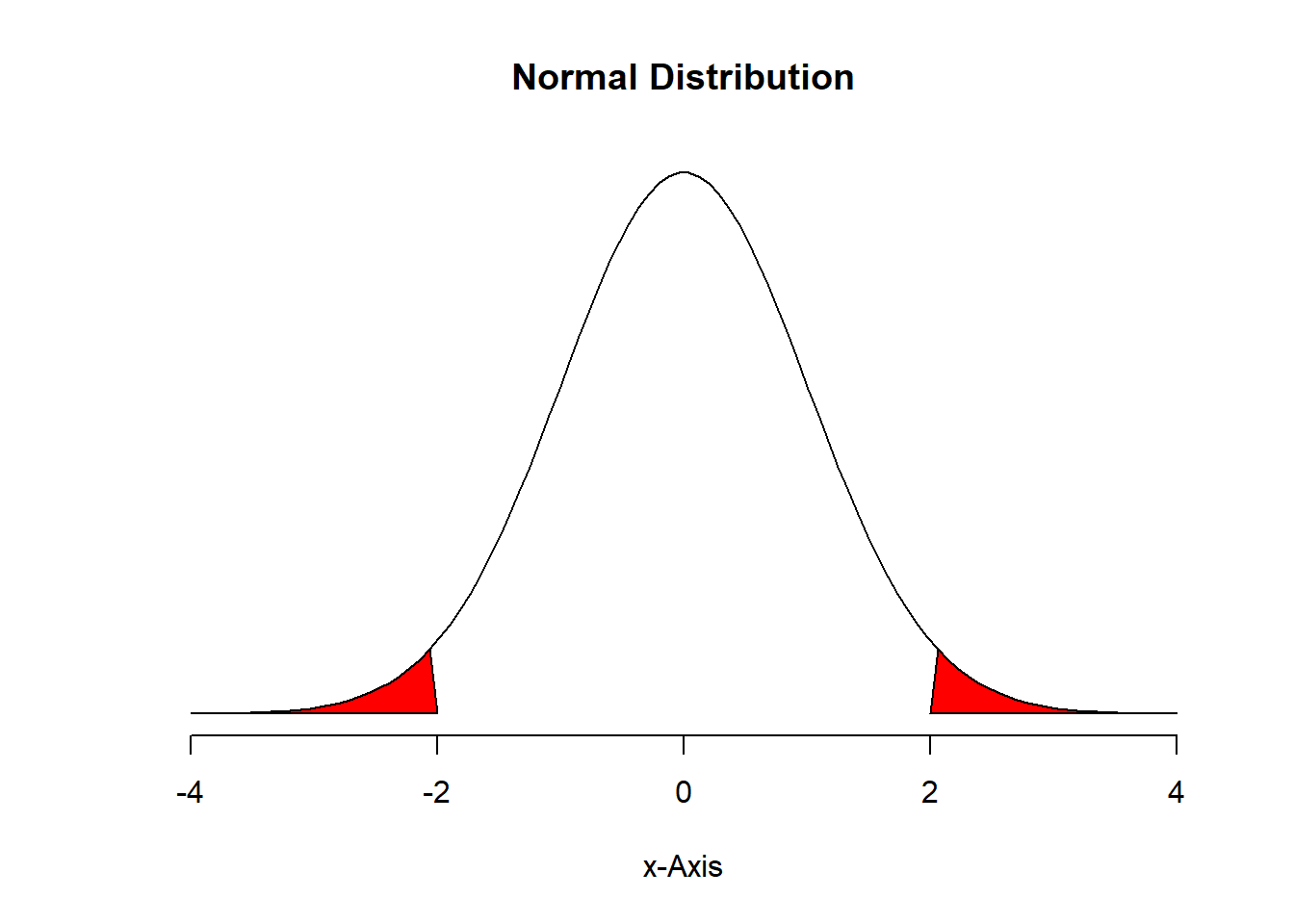The percent of a standard normal distribution found in this region is: 4.55%

Triathlon times, Part I (4.4, p. 142) In triathlons, it is common for racers to be placed into age and gender groups. Friends Leo and Mary both completed the Hermosa Beach Triathlon, where Leo competed in the Men, Ages 30 - 34 group while Mary competed in the Women, Ages 25 - 29 group. Leo completed the race in 1:22:28 (4948 seconds), while Mary completed the race in 1:31:53 (5513 seconds). Obviously Leo finished faster, but they are curious about how they did within their respective groups. Can you help them? Here is some information on the performance of their groups:

• The finishing times of the group has a mean of 4313 seconds with a standard deviation of 583 seconds.
• The finishing times of the group has a mean of 5261 seconds with a standard deviation of 807 seconds.
• The distributions of finishing times for both groups are approximately Normal.

Remember: a better performance corresponds to a faster finish.

## (a) Write down the short-hand for these two normal distributions.

For Men (Leo’s Group): N(µ = 4313, σ = 583)

For Women (Mary’s Group): N(µ = 5261, σ = 807)

## (b) What are the Z-scores for Leo’s and Mary’s finishing times? What do these Z-scores tell you?

Z_Leo = (4948 - 4313)/583 = 1.09

Z_Mary = (5513 - 5261)/807 = 0.31

Z_Leo <- (4948 - 4313)/583

Z_Mary <- (5513 - 5261)/807

Z_Leo
##  1.089194
Z_Mary
##  0.3122677

Leo is 1.09 standard deviations slower than the mean for his men group. Mary is 0.31 standard deviations slower than the mean for her women group.

## (c) Did Leo or Mary rank better in their respective groups? Explain your reasoning.

Answer: Mary was faster with respect to her group because of her Z-score which was lower, since lower Z-scores means faster times. So Mary did rank better than Leo; only 13.8% were slower than Leo, but 37.8% were slower than Mary (see following calculations).

#for Leo
1-pnorm(4948, mean = 4313, sd=583)
##  0.1380342
#for Mary
1-pnorm(5513, mean = 5261, sd=807)
##  0.3774186
1. What percent of the triathletes did Leo finish faster than in his group?

Answer: Leo finished faster than 13.8% of triathletes.

#for Leo
1 - pnorm(Z_Leo, mean = 0, sd = 1)
##  0.1380342
1. What percent of the triathletes did Mary finish faster than in her group?

Answer: Mary finished faster than 37.7% of triathletes.

#for Mary
1 - pnorm(Z_Mary, mean = 0, sd = 1)
##  0.3774186
1. If the distributions of finishing times are not nearly normal, would your answers to parts (b) - (e) change? Explain your reasoning.

Answer: The answer to (b) will be the same, but the other answers will change, because for the other distributions, the percentiles are not the same.

Heights of female college students Below are heights of 25 female college students.

$\stackrel{1}{54}, \stackrel{2}{55}, \stackrel{3}{56}, \stackrel{4}{56}, \stackrel{5}{57}, \stackrel{6}{58}, \stackrel{7}{58}, \stackrel{8}{59}, \stackrel{9}{60}, \stackrel{10}{60}, \stackrel{11}{60}, \stackrel{12}{61}, \stackrel{13}{61}, \stackrel{14}{62}, \stackrel{15}{62}, \stackrel{16}{63}, \stackrel{17}{63}, \stackrel{18}{63}, \stackrel{19}{64}, \stackrel{20}{65}, \stackrel{21}{65}, \stackrel{22}{67}, \stackrel{23}{67}, \stackrel{24}{69}, \stackrel{25}{73}$

1. The mean height is 61.52 inches with a standard deviation of 4.58 inches. Use this information to determine if the heights approximately follow the 68-95-99.7% Rule.

pnorm(66.1, mean=61.52, sd=4.58) - pnorm(56.94, mean=61.52, sd=4.58)
##  0.6826895
pnorm(70.68, mean=61.52, sd=4.58) - pnorm(52.36, mean=61.52, sd=4.58)
##  0.9544997
pnorm(75.26, mean=61.52, sd=4.58) - pnorm(47.78, mean=61.52, sd=4.58)
##  0.9973002
1. Do these data appear to follow a normal distribution? Explain your reasoning using the graphs provided below.

Answer: Yes, the data seem to follow the normal distribution. the histogram does not look so symetric like its supposed to be, but the the Bell curve is a good approximation. The quantiles plot is close to a line, which what must happen if it were normally distributed.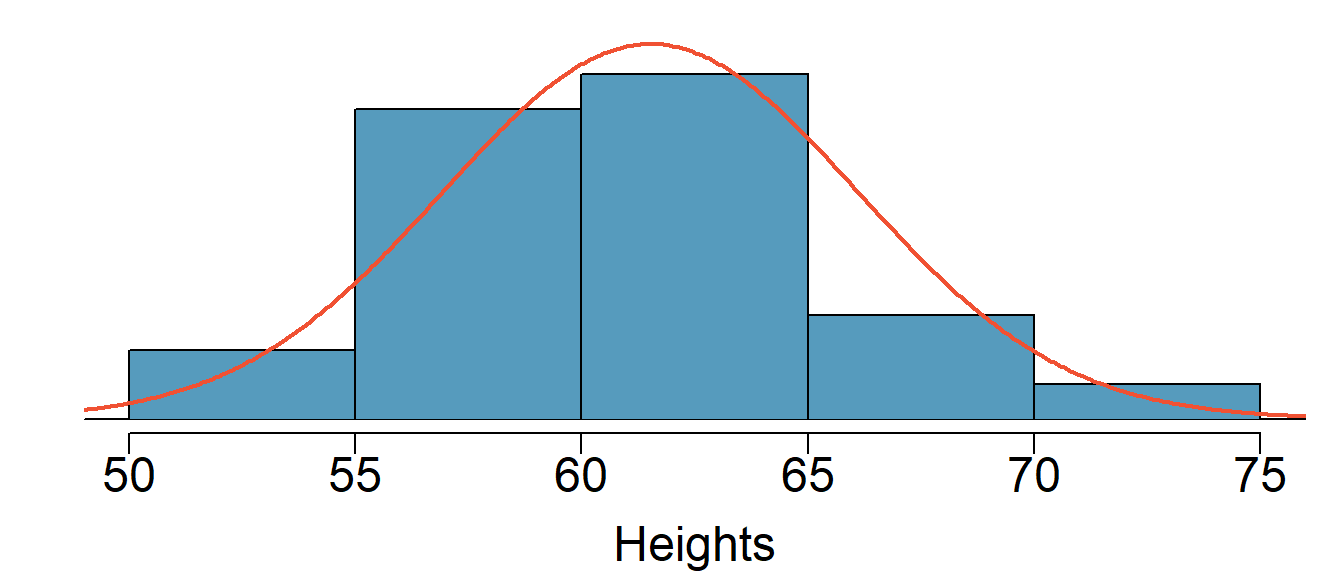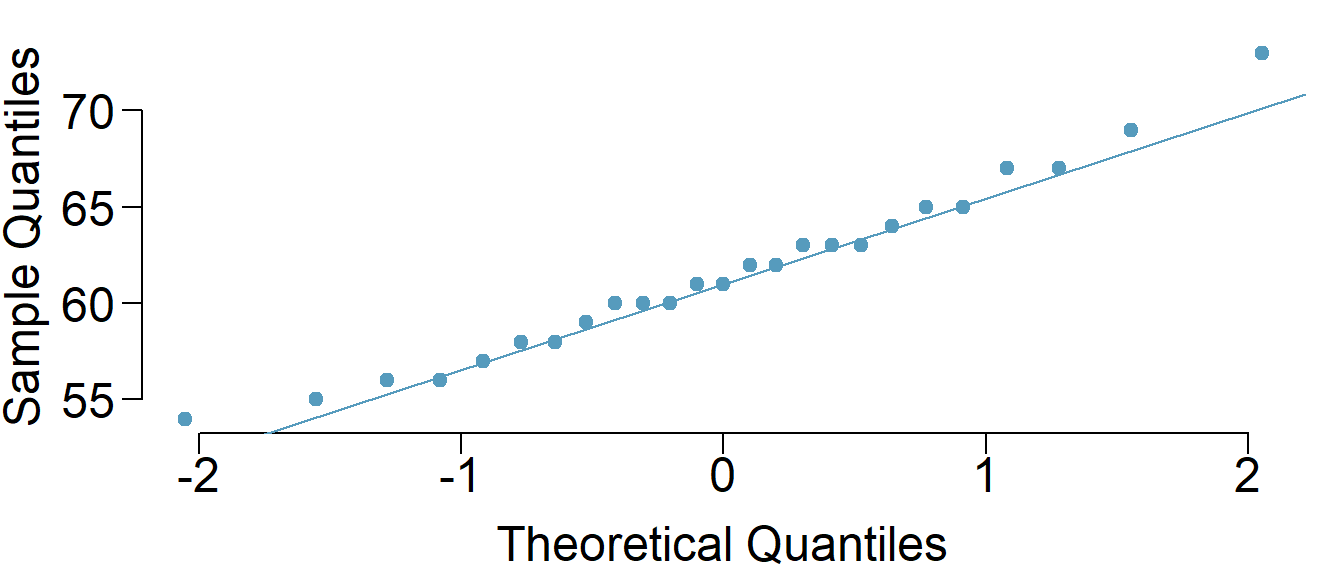# Use the DATA606::qqnormsim function

Defective rate. (4.14, p. 148) A machine that produces a special type of transistor (a component of computers) has a 2% defective rate. The production is considered a random process where each transistor is independent of the others.

1. What is the probability that the 10th transistor produced is the first with a defect?
p <- (1-.02)^(10-1)*.02
p
##  0.01667496
1. What is the probability that the machine produces no defective transistors in a batch of 100?
p <- (1-.02)^100
p
##  0.1326196
1. On average, how many transistors would you expect to be produced before the first with a defect? What is the standard deviation?
# expected value = e.v = 1/p
e.v <- 1/.02
e.v
##  50
# sd = sqrt((1-p))/p*p)
sd <- sqrt((1-.02)/(.02*.02))
sd
##  49.49747
1. Another machine that also produces transistors has a 5% defective rate where each transistor is produced independent of the others. On average how many transistors would you expect to be produced with this machine before the first with a defect? What is the standard deviation?
# expected value = e.v = 1/p
e.v <- 1/.05
e.v
##  20
# sd = sqrt((1-p))/p*p)
sd <- sqrt((1-.05)/(.05*.05))
sd
##  19.49359
1. Based on your answers to parts (c) and (d), how does increasing the probability of an event affect the mean and standard deviation of the wait time until success?

Answer: when the probability of failure increases, implies that the event is more common. So both the expected number of trials before a success and the standard deviation of waiting time will decrease.

Male children. While it is often assumed that the probabilities of having a boy or a girl are the same, the actual probability of having a boy is slightly higher at 0.51. Suppose a couple plans to have 3 kids.

1. Use the binomial model to calculate the probability that two of them will be boys.

Answer: the probability that two of them will be boys is 0.38.

pb <- .51
p = dbinom(2,3,pb)
p
##  0.382347
1. Write out all possible orderings of 3 children, 2 of whom are boys. Use these scenarios to calculate the same probability from part (a) but using the addition rule for disjoint outcomes. Confirm that your answers from parts (a) and (b) match.

Answer: the possiblities are: gbb, bbg and bgb

# so p = P(gbb) + P(bbg) + P(bgb)
p <- (.49 * .51 * .51) + (.51 * .51 * .49) + (.51 * .49 * .51)
p
##  0.382347
1. If we wanted to calculate the probability that a couple who plans to have 8 kids will have 3 boys, briefly describe why the approach from part (b) would be more tedious than the approach from part (a).

Answer: More kids they want to have, more combinations would be. In case of planning to have 8 kids, they will be 56 combinations, and to do all the calculations using the addition rule like in (b), will be tedious, laborious and error prone. But if we use the (a) approach, it’s just one line formula where we plug the numbers.

Serving in volleyball. (4.30, p. 162) A not-so-skilled volleyball player has a 15% chance of making the serve, which involves hitting the ball so it passes over the net on a trajectory such that it will land in the opposing team’s court. Suppose that her serves are independent of each other.

1. What is the probability that on the 10th try she will make her 3rd successful serve?

Answer: the probability that on the 10th try she will make her 3rd successful serve is 0.039 .

p_ms <- .15
n <- 10
k <- 3
p <- choose(n-1, k-1) * (1-p_ms)^(n-k)*p_ms^k
p
##  0.03895012
1. Suppose she has made two successful serves in nine attempts. What is the probability that her 10th serve will be successful?

Answer: Since all her serves are independent of each others, the probablity that her 10th serve will be successful will still be 0.15 .

1. Even though parts (a) and (b) discuss the same scenario, the probabilities you calculated should be different. Can you explain the reason for this discrepancy?

Answer: (a) is dealing with a join probability, while (b) is dealing with a single independent trial (the probability that her 10th trail will be suceessful).